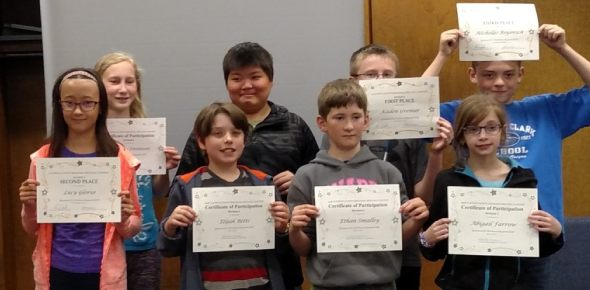10 Questions | Total Attempts: 89SettingsGreek and latin root word list : quad-

• 1.
• A.

Four sided enclosure, usually surrounded by building

• B.

Collection or group of our: one of four babies born from the same mother at the same time

• C.

An animal with four feet

• D.

Happening every four years

• 2.
• A.

To cut or divide into four equal parts

• B.

One out of four equal parts of a circle: one section of a four-section coordinate grid

• C.

The ability tto speak four languages

• D.

A four -sided enclosure, usually surrounded by building

• 3.
• A.

To cut or divide into four equal parts

• B.

Happening every four years

• C.

To create four times as much or as many of something

• D.

The ability to speak four languages

• 4.
• A.

A plane figure in qeometry that has four sides

• B.

An animal with four feet

• C.

A muscle group consisting of four muscles that is located along tthe front of the thigh

• D.

To create four times as much or as many of something

• 5.
• A.

One out of four equal parts of a circle, one section of a four-section coordinate grid

• B.

The ability to speak four languages

• C.

To cut or divide into four equal parts

• D.

A plane figure in geometry that has four sides

• 6.
• A.

Happening every four years

• B.

An animal with four feet

• C.

The ability to speak four languages

• D.

To create four times as much or as many of something

• 7.
• A.

To cut or divide into four equal parts

• B.

One out of four equal parts of a circle, one section of a four-section coordinate grid

• C.

Four muscles that is located along the front of the thigh

• D.

An animal with four feet

• 8.
• A.

A collection or group of four; one of four babies born from the same mother at the same time

• B.

Happening every four years

• C.

An animal with four feet

• D.

On out of four equal parts of a circle; one section of a four-section coordinate grid

• 9.
• A.

To create four times as much or as many of something

• B.

A plane figure in geometry that has four sides

• C.

A collection or group of four; one of four babies born from the same mother at the same time

• D.

To cut or divide into four equal parts

• 10.
• A.

Happenig every for years

• B.

The ability to speak four languages

• C.

A collection or group of four; one of four babies born from the same mother atthe same time

• D.

One out of four equal parts of a circle, one section of a four-section coordinate grid

Related TopicsBack to top